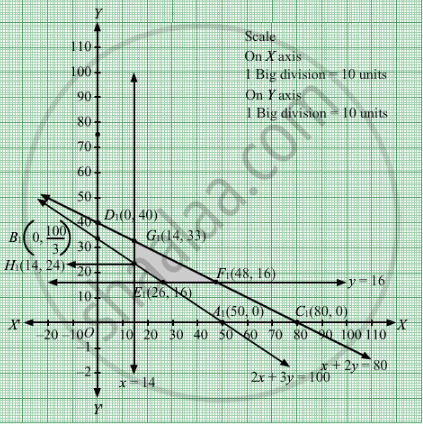# A Manufacturer Makes Two Products a and B. Product a Sells at Rs 200 Each and Takes 1/2 Hour to Make. Product B Sells at Rs 300 Each and Takes 1 Hour to Make. - Mathematics

Sum

A manufacturer makes two products A and B. Product A sells at Rs 200 each and takes 1/2 hour to make. Product B sells at Rs 300 each and takes 1 hour to make. There is a permanent order for 14 of product A and 16 of product B. A working week consists of 40 hours of production and weekly turnover must not be less than Rs 10000. If the profit on each of product A is Rs 20 and on product B is Rs 30, then how many of each should be produced so that the profit is maximum. Also, find the maximum profit.

#### Solution

Let x units of product A and y units of product B were manufactured.
Number of units cannot be negative.
Therefore, $x, y \geq 0$

According to question, the given information can be tabulated as:

 Selling price(Rs) Manufacturing time(hrs) Product A(x) 200 0.5 Product B(y) 300 1

Also, the availability of time is 40 hours and the revenue should be atleast Rs 10000.

Further, it is given that there is a permanent order for 14 units of product A and 16 units of product B.

Therefore, the constraints are

$200x + 300y \geq 10000$

$0 . 5x + y \leq 40$

$x \geq 14$

$y \geq 16$

If the profit on each of product A is Rs 20 and on product B is Rs 30.Therefore, profit gained on x units of product A and y units of product is Rs 20x and Rs 30y respectively.

Total profit = Z = $20x + 30y$which is to be maximised
Thus, the mathematical formulat​ion of the given linear programmimg problem is
Max Z =  $20x + 30y$

subject to

$2x + 3y \geq 100$
$x + 2y \leq 80$
$x \geq 14$
$y \geq 16$

$x, y \geq 0$

First we will convert inequations into equations as follows:
2x + 3y = 100, x + 2y = 80, x = 14, y = 16, x = 0 and y = 0.
Region represented by 2x + 3y ≥ 100:
The line 2x + 3y = 100 meets the coordinate axes at A1(50, 0) and $B_1 \left( 0, \frac{100}{3} \right)$ respectively. By joining these points we obtain the line 2x + 3y = 100 .

Clearly (0,0) does not satisfies the 2x + 3y = 100. So, the region which does not contain the origin represents the solution set of the inequation 2x + 3y ≥ 100.

Region represented by x + 2y ≤ 80:
The line x + 2y = 80 meets the coordinate axes at C1(80, 0) and D1(0, 40) respectively. By joining these points we obtain the line
x + 2y = 80. Clearly (0,0) satisfies the inequation x + 2y ≤ 80. So,the region which contains the origin represents the solution set of the inequation x + 2y ≤ 80.

Region represented by x ≥ 14
x = 14 is the line passes through (14, 0) and is parallel to the Y axis.The region to the right of the line x = 14 will satisfy the inequation.

Region represented by y ≥ 16
y = 16 is the line passes through (0, 16) and is parallel to the X axis.The region above the line y = 16 will satisfy the inequation.

Region represented by ≥ 0 and y ≥ 0:
Since, every point in the first quadrant satisfies these inequations. So, the first quadrant is the region represented by the inequations x ≥ 0, and y ≥ 0.

The feasible region determined by the system of constraints 2x + 3y ≥ 100, x + 2y ≤ 80, x≥ 14, y ≥ 16, x ≥ 0 and y ≥ 0 are as follows.The corner points of the feasible region are E1(26, 16), F1(48, 16), G1(14, 33) and H1(14, 24)

The values of Z at these corner points are as follows

 Corner point Z= 20x + 30y E1 1000 F1 1440 G1 1270 H1 1000

The maximum value of Z is Rs 1440 which is attained at F1

$\left( 48, 16 \right)$
Thus, the maximum profit is Rs 1440 obtained when 48 units of product A and 16 units of product were manufactured.

Concept: Graphical Method of Solving Linear Programming Problems
Is there an error in this question or solution?

#### APPEARS IN

RD Sharma Class 12 Maths
Chapter 30 Linear programming
Exercise 30.4 | Q 19 | Page 53